Next: Motion of a Submerged Up: Axisymmetric Incompressible Inviscid Flow Previous: Dipole Point Sources

# Flow Past a Spherical Obstacle

Consider the steady flow pattern produced when an impenetrable rigid spherical obstacle is placed in a uniformly flowing, incompressible, inviscid fluid. For instance, suppose that the radius of the sphere is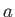, and that its center coincides with the origin. Furthermore, let the unperturbed fluid velocity be of magnitude, and be directed parallel to the-axis. We expect the flow pattern to remain unperturbed very far away from the sphere. In other words, we expect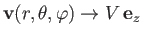as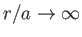. Given that the fluid velocity field a large distance upstream of the sphere is irrotational (because a uniform flow pattern is automatically irrotational), it follows from the Kelvin circulation theorem that the velocity field remains irrotational as it is convected past the sphere. (See Section 4.14.) Hence, we can write, where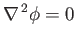(7.45)

(because the fluid is incompressible.) The boundary conditions are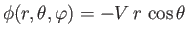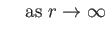(7.46)

and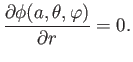(7.47)

The latter constraint arises because the surface of the sphere is impenetrable, which implies that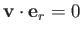at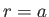.

Let us search for an axisymmetric solution of Equation (7.45) of the form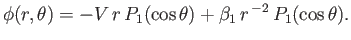(7.48)

It can be seen, by comparison with Equation (7.23), that the previous expression definitely solves Equation (7.45). Moreover, the expression also automatically satisfies the boundary condition (7.46) [because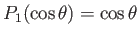]. The remaining boundary condition, (7.47), yields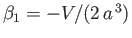. Hence, we obtain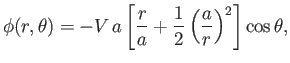(7.49)

or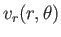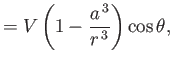(7.50)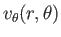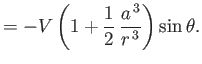(7.51)

Because the solutions of Laplace's equation, subject to well-posed boundary conditions, are unique (Riley 1974), we can be sure that the previous axisymmetric solution is the most general solution to the problem.

It is clear, by comparison with Equations (7.28) and (7.42), that the velocity potential (7.49) is the superposition of that associated with uniform flow with velocity, parallel to the-axis, and a dipole point source of strength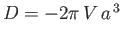, located at the origin. Thus, making use of Equations (7.26) and (7.44), the associated stream function takes the form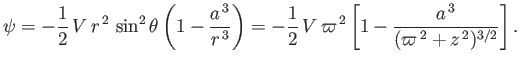(7.52)

Figure 7.4 show the contours of this stream function.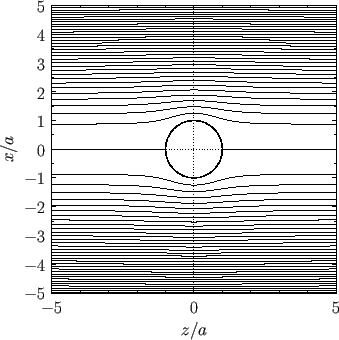Bernoulli's theorem yields (see Section 4.3)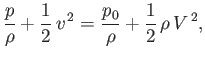(7.53)

whereis the uniform fluid mass density, and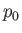the fluid pressure at infinity. Thus, making use of Equations (7.50) and (7.51), the pressure distribution on the surface of the sphere can be written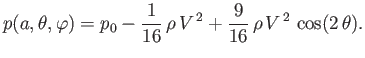(7.54)

The net force exerted on the sphere by the fluid has the Cartesian components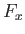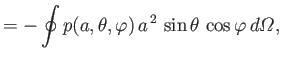(7.55)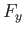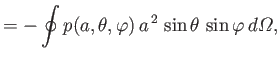(7.56)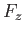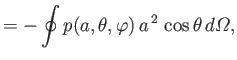(7.57)

where the integrals are over all solid angle. Thus, it follows that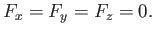(7.58)

In other words, the fluid exerts zero net force on the sphere, in accordance with d'Alembert's paradox. (See Section 4.5.)Next: Motion of a Submerged Up: Axisymmetric Incompressible Inviscid Flow Previous: Dipole Point Sources
Richard Fitzpatrick 2016-03-31